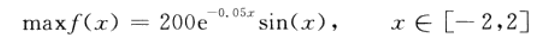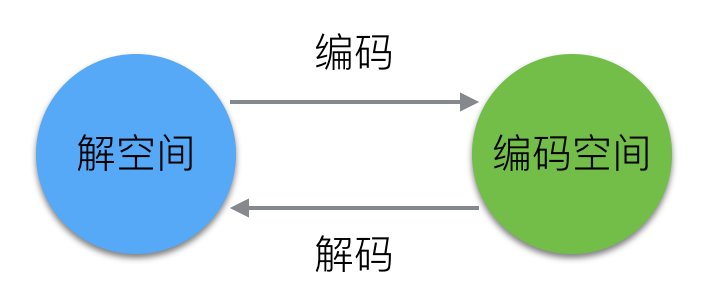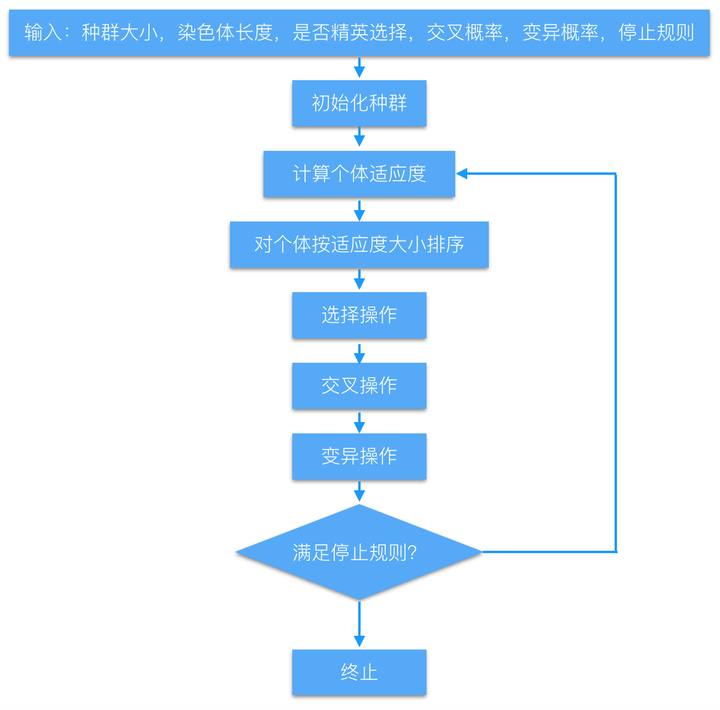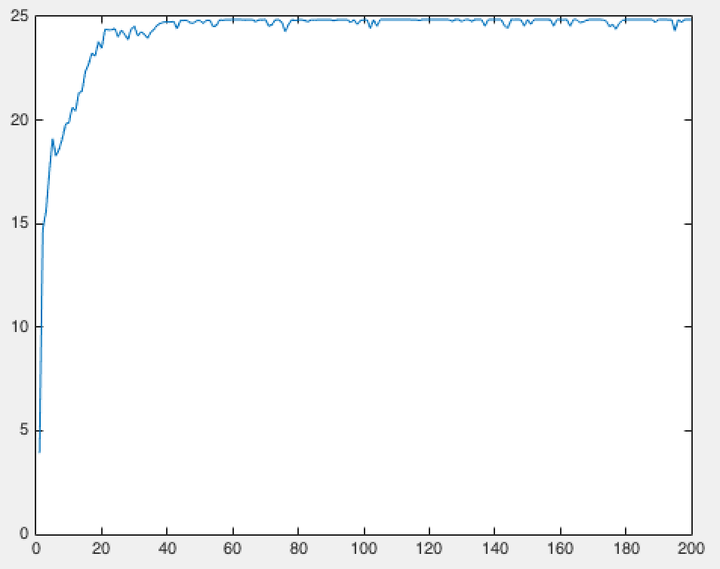• 遗传算法的题目和解答（Matlab代码） 题目：用标准遗传算法求解函数的最大值，其中的取值范围是。 解答：
遗传算法的题目和解答（Matlab代码）
题目：用标准遗传算法求解函数f(x)=x+10sin(5x)+7cos(4x)的最大值，其中x的取值范围是[0,10]。 解答：优化结束后，根据适应度曲线的图，可以得出优化结果为x=7.8567,
clear all; %清除所有变量
close all; %清图
clc;    %清屏
NP=50;  %种群数量
L=20;   %二进制位串长度
Pc=0.8; %交叉率
Pm=0.1; %变异率
G=100;  %最大遗传代数
Xs=10;  %上限
Xx=0;   %下限
f=randi(NP,L);  %随机获得初始种群
% 遗传算法循环
for k=1:G	%将二进制解码为定义域范围内十进制
for i=1:NP
U=f(i,:);
m=0;
for j=1:L
m=U(j)*2^(j-1)+m;
end
x(i)=Xx+m*(Xx-Xx)/(2^L-1);
Fit(i)=func1(x(i));
end
maxFit=max(Fit);	%最大值
minFit=min(Fit);	%最小值
rr=find(Fit==maxFit);
fBest=f(rr(1,1),:);	  %历代最优个体
xBest=x(rr(1,1));
Fit=(Fit-minFit)/(maxFit-minFit);	%归一化适应度值
%基于轮盘赌的复制操作
sum_Fit=sum(Fit);
fitvalue=Fit./sum_Fit;
fitvalue=cumsum(fitvalue);
ms=sort(rand(NP,1));
fiti=1;
newi=1;
while newi<=NP
if(ms(newi))<fitvalue(fiti)
nf(newi,:)=f(fiti,:);
newi=newi+1;
else
fiti=fiti+1;
end
end
%交叉操作
for i=1:2:NP
p=rand;
if p<Pc
q=randi(1,L);
for j=1:L
if q(j)==1;
temp=nf(i+1,j);
nf(i+1,j)=nf(i,j);
nf(i,j)=temp;
end
end
end
end
%变异操作
i=1;
while i<=rand(NP*Nm)
h=randi(1,1,[1,NP]);
for j=1:round(L*Pm)
g=randi(1,1,[1,L]);
nf(h,g)=~nf(h,g);
end
i=i+1;
end
f=nf;
f(1,:)=fBest;       %保留最优个体在新种群中
trace(k)=maxFit;    %历代最优适应度
end
xBest;  %最优个体
figure;
plot(trace);
xlabel('迭代次数');
ylabel('目标函数值');
title('适应度进化曲线');

function result=func1(x)
fit=x+10*sin(5*x)+7*cos(4*x);
result=fit;
end

展开全文• 遗传算法（Genetic Algorithm）是一类借鉴生物界的进化规律（适者生存，优胜劣汰遗传机制）演化而来的随机化搜索方法。它是由美国的J.Holland教授1975年首先提出，其主要特点是直接对结构对象进行操作，不存在求导和...
• 遗传算法求解在[-2 2]区间上的最大值 2、主程序： %主程序：用遗传算法求解y=200*exp(-0.05*x).*sin(x)在[-2 2]区间上的最大值 clc; clear all; close all; global BitLength global boundsbegin global boundsend...
1、题目
用遗传算法求解在[-2 2]区间上的最大值
2、主程序：
%主程序：用遗传算法求解y=200*exp(-0.05*x).*sin(x)在[-2 2]区间上的最大值
clc;
clear all;
close all;
global BitLength
global boundsbegin
global boundsend
bounds=[-2 2];%一维自变量的取值范围
precision=0.0001; %运算精度
boundsbegin=bounds(:,1);
boundsend=bounds(:,2);
%计算如果满足求解精度至少需要多长的染色体
BitLength=ceil(log2((boundsend-boundsbegin)' ./ precision));
popsize=50; %初始种群大小
Generationnmax=12;  %最大代数
pcrossover=0.90; %交配概率
pmutation=0.09; %变异概率
%产生初始种群
population=round(rand(popsize,BitLength));
%计算适应度,返回适应度Fitvalue和累积概率cumsump
[Fitvalue,cumsump]=fitnessfun(population);          %子程序1
Generation=1;
while Generation<Generationnmax+1
for j=1:2:popsize
%选择操作
seln=selection(population,cumsump);          % 子程序2
%交叉操作
scro=crossover(population,seln,pcrossover);            %子程序3
scnew(j,:)=scro(1,:);
scnew(j+1,:)=scro(2,:);
%变异操作
smnew(j,:)=mutation(scnew(j,:),pmutation);                    %子程序4
smnew(j+1,:)=mutation(scnew(j+1,:),pmutation);
end
population=smnew;  %产生了新的种群
%计算新种群的适应度
[Fitvalue,cumsump]=fitnessfun(population);
%记录当前代最好的适应度和平均适应度
[fmax,nmax]=max(Fitvalue);
fmean=mean(Fitvalue);
ymax(Generation)=fmax;
ymean(Generation)=fmean;
%记录当前代的最佳染色体个体
x=transform2to10(population(nmax,:));              %子程序5
%自变量取值范围是[-2 2],需要把经过遗传运算的最佳染色体整合到[-2 2]区间
xx=boundsbegin+x*(boundsend-boundsbegin)/(power((boundsend),BitLength)-1);
xmax(Generation)=xx;
Generation=Generation+1
end
Generation=Generation-1;
Bestpopulation=xx
Besttargetfunvalue=targetfun(xx)                  %子程序6
%绘制经过遗传运算后的适应度曲线。一般地，如果进化过程中种群的平均适应度与最大适
%应度在曲线上有相互趋同的形态，表示算法收敛进行得很顺利，没有出现震荡；在这种前
%提下，最大适应度个体连续若干代都没有发生进化表明种群已经成熟。
figure(1);
hand1=plot(1:Generation,ymax);
set(hand1,'linestyle','-','linewidth',1.8,'marker','*','markersize',6)
hold on;
hand2=plot(1:Generation,ymean);
set(hand2,'color','r','linestyle','-','linewidth',1.8,...
'marker','h','markersize',6)
xlabel('进化代数');ylabel('最大/平均适应度');xlim([1 Generationnmax]);
legend('最大适应度','平均适应度');
box off;hold off;

%子程序1：计算适应度函数, 函数名称存储为fitnessfun
function [Fitvalue,cumsump]=fitnessfun(population);
global BitLength
global boundsbegin
global boundsend
popsize=size(population,1);   %有popsize个个体
for i=1:popsize
x=transform2to10(population(i,:));  %将二进制转换为十进制
%转化为[-2,2]区间的实数
xx=boundsbegin+x*(boundsend-boundsbegin)/(power((boundsend),BitLength)-1);
Fitvalue(i)=targetfun(xx);  %计算函数值，即适应度
end
%给适应度函数加上一个大小合理的数以便保证种群适应值为正数
Fitvalue=Fitvalue'+230;
%计算选择概率
fsum=sum(Fitvalue);
Pperpopulation=Fitvalue/fsum;
%计算累积概率
cumsump(1)=Pperpopulation(1);
for i=2:popsize
cumsump(i)=cumsump(i-1)+Pperpopulation(i);
end
cumsump=cumsump';

%子程序2：新种群选择操作, 函数名称存储为selection.m
function seln=selection(population,cumsump);
%从种群中选择两个个体
for i=1:2
r=rand;  %产生一个随机数
prand=cumsump-r;
j=1;
while prand(j)<0
j=j+1;
end
seln(i)=j; %选中个体的序号
end

%子程序3：新种群交叉操作,函数名称存储为crossover.m
function scro=crossover(population,seln,pc);
BitLength=size(population,2);
pcc=IfCroIfMut(pc);  %根据交叉概率决定是否进行交叉操作，1则是，0则否
if pcc==1
chb=round(rand*(BitLength-2))+1;  %在[1,BitLength-1]范围内随机产生一个交叉位
scro(1,:)=[population(seln(1),1:chb) population(seln(2),chb+1:BitLength)];
scro(2,:)=[population(seln(2),1:chb) population(seln(1),chb+1:BitLength)];
else
scro(1,:)=population(seln(1),:);
scro(2,:)=population(seln(2),:);
end

%子程序4：新种群变异操作，函数名称存储为mutation.m
function snnew=mutation(snew,pmutation);
BitLength=size(snew,2);
snnew=snew;
pmm=IfCroIfMut(pmutation);  %根据变异概率决定是否进行变异操作，1则是，0则否     %子程序7
if pmm==1
chb=round(rand*(BitLength-1))+1;  %在[1,BitLength]范围内随机产生一个变异位
snnew(chb)=abs(snew(chb)-1);
end

%子程序7：判断遗传运算是否需要进行交叉或变异, 函数名称存储为IfCroIfMut.m
function pcc=IfCroIfMut(mutORcro);
test(1:100)=0;
l=round(100*mutORcro);
test(1:l)=1;
n=round(rand*99)+1;
pcc=test(n);

%子程序5：将2进制数转换为10进制数,函数名称存储为transform2to10.m
function x=transform2to10(Population);
BitLength=size(Population,2);
x=Population(BitLength);
for i=1:BitLength-1
x=x+Population(BitLength-i)*power(2,i);
end

%子程序6：对于优化最大值或极大值函数问题，目标函数可以作为适应度函数
%函数名称存储为targetfun.m
function y=targetfun(x); %目标函数
y=200*exp(-0.05*x).*sin(x);           %可更改！！


展开全文• 自从遗传算法被提出以来，其得到了广泛的应用，特别是在函数优化、生产调度、模式识别、神经网络、自适应控制等领域，遗传算法发挥了很大的作用，提高了一些问题求解的效率。 2.遗传算法组成 编码 ->...
作者：sjyan
来源：知乎
著作权归作者所有。商业转载请联系作者获得授权，非商业转载请注明出处。

求解函数 f(x) = x + 10*sin(5*x) + 7*cos(4*x) 在区间[0,9]的最大值。那么如何应用遗传算法如何来找到这个奇怪的函数的最大值呢？
事实上，不管一个函数的形状多么奇怪，遗传算法都能在很短的时间内找到它在一个区间内的(近似)最大值。
相当神奇，不是吗？
接下来围绕这个问题，讲讲我对遗传算法的一些理解。实现代码以及在Matlab中使用遗传算法的小教程都附在最后。

1.介绍
遗传算法(Genetic Algorithm)遵循『适者生存』、『优胜劣汰』的原则，是一类借鉴生物界自然选择和自然遗传机制的随机化搜索算法。
遗传算法模拟一个人工种群的进化过程，通过选择(Selection)、交叉(Crossover)以及变异(Mutation)等机制，在每次迭代中都保留一组候选个体，重复此过程，种群经过若干代进化后，理想情况下其适应度达到***近似最优***的状态。
自从遗传算法被提出以来，其得到了广泛的应用，特别是在函数优化、生产调度、模式识别、神经网络、自适应控制等领域，遗传算法发挥了很大的作用，提高了一些问题求解的效率。

2.遗传算法组成
编码 -> 创造染色体个体 -> 种群适应度函数遗传算子
选择交叉变异

运行参数
是否选择精英操作种群大小染色体长度最大迭代次数交叉概率变异概率

2.1 编码与解码
实现遗传算法的第一步就是明确对求解问题的编码和解码方式。
对于函数优化问题，一般有两种编码方式，各具优缺点
实数编码：直接用实数表示基因，容易理解且不需要解码过程，但容易过早收敛，从而陷入局部最优二进制编码：稳定性高，种群多样性大，但需要的存储空间大，需要解码且难以理解

对于求解函数最大值问题，我选择的是二进制编码。 &amp;lt;img src=&quot;https://pic4.zhimg.com/50/6f49b2e302fbebe4d3c4242495e3b1ab_hd.jpg&quot; data-caption=&quot;&quot; data-size=&quot;normal&quot; data-rawwidth=&quot;706&quot; data-rawheight=&quot;304&quot; class=&quot;origin_image zh-lightbox-thumb&quot; width=&quot;706&quot; data-original=&quot;https://pic4.zhimg.com/6f49b2e302fbebe4d3c4242495e3b1ab_r.jpg&quot;&amp;gt;以我们的目标函数 f(x) = x + 10sin(5x) + 7cos(4x), x∈[0,9] 为例。
假如设定求解的精度为小数点后4位，可以将x的解空间划分为 (9-0)×(1e+4)=90000个等分。
2^16<90000<2^17，需要17位二进制数来表示这些解。换句话说，一个解的编码就是一个17位的二进制串。
一开始，这些二进制串是随机生成的。
一个这样的二进制串代表一条染色体串，这里染色体串的长度为17。
对于任何一条这样的染色体chromosome，如何将它复原(解码)到[0,9]这个区间中的数值呢？
对于本问题，我们可以采用以下公式来解码：

x = 0 + decimal(chromosome)×(9-0)/(2^17-1)


decimal( ): 将二进制数转化为十进制数
一般化解码公式：

f(x), x∈[lower_bound, upper_bound]
x = lower_bound + decimal(chromosome)×(upper_bound-lower_bound)/(2^chromosome_size-1)


lower_bound: 函数定义域的下限 upper_bound: 函数定义域的上限 chromosome_size: 染色体的长度
通过上述公式，我们就可以成功地将二进制染色体串解码成[0,9]区间中的十进制实数解。
2.2 个体与种群
『染色体』表达了某种特征，这种特征的载体，称为『个体』。
对于本次实验所要解决的一元函数最大值求解问题，个体可以用上一节构造的染色体表示，一个个体里有一条染色体。
许多这样的个体组成了一个种群，其含义是一个一维点集(x轴上[0,9]的线段)。
2.3 适应度函数
遗传算法中，一个个体(解)的好坏用适应度函数值来评价，在本问题中，f(x)就是适应度函数。
适应度函数值越大，解的质量越高。
适应度函数是遗传算法进化的驱动力，也是进行自然选择的唯一标准，它的设计应结合求解问题本身的要求而定。
2.4 遗传算子
我们希望有这样一个种群，它所包含的个体所对应的函数值都很接近于f(x)在[0,9]上的最大值，但是这个种群一开始可能不那么优秀，因为个体的染色体串是随机生成的。
如何让种群变得优秀呢？
不断的进化。
每一次进化都尽可能保留种群中的优秀个体，淘汰掉不理想的个体，并且在优秀个体之间进行染色体交叉，有些个体还可能出现变异。
种群的每一次进化，都会产生一个最优个体。种群所有世代的最优个体，可能就是函数f(x)最大值对应的定义域中的点。
如果种群无休止地进化，那总能找到最好的解。但实际上，我们的时间有限，通常在得到一个看上去不错的解时，便终止了进化。
对于给定的种群，如何赋予它进化的能力呢？
首先是选择(selection)
选择操作是从前代种群中选择***多对***较优个体，一对较优个体称之为一对父母，让父母们将它们的基因传递到下一代，直到下一代个体数量达到种群数量上限在选择操作前，将种群中个体按照适应度从小到大进行排列采用轮盘赌选择方法（当然还有很多别的选择方法），各个个体被选中的概率与其适应度函数值大小成正比轮盘赌选择方法具有随机性，在选择的过程中可能会丢掉较好的个体，所以可以使用精英机制，将前代最优个体直接选择

其次是交叉(crossover)
两个待交叉的不同的染色体(父母)根据交叉概率(cross_rate)按某种方式交换其部分基因采用单点交叉法，也可以使用其他交叉方法

最后是变异(mutation)
染色体按照变异概率(mutate_rate)进行染色体的变异采用单点变异法，也可以使用其他变异方法

一般来说，交叉概率(cross_rate)比较大，变异概率(mutate_rate)极低。像求解函数最大值这类问题，我设置的交叉概率(cross_rate)是0.6，变异概率(mutate_rate)是0.01。
因为遗传算法相信2条优秀的父母染色体交叉更有可能产生优秀的后代，而变异的话产生优秀后代的可能性极低，不过也有存在可能一下就变异出非常优秀的后代。这也是符合自然界生物进化的特征的。

3.遗传算法流程 &amp;lt;img src=&quot;https://pic2.zhimg.com/50/bbe28bbf296e4762e64867314b90bca3_hd.jpg&quot; data-caption=&quot;&quot; data-size=&quot;normal&quot; data-rawwidth=&quot;1202&quot; data-rawheight=&quot;1186&quot; class=&quot;origin_image zh-lightbox-thumb&quot; width=&quot;1202&quot; data-original=&quot;https://pic2.zhimg.com/bbe28bbf296e4762e64867314b90bca3_r.jpg&quot;&amp;gt;附上实现代码:  genetic-algorithm
其中包含了遗传算法的 Matlab 实现和 Tensorflow 实现 (开发中)。

测试结果
最优个体：00011111011111011最优适应度：24.8554最优个体对应自变量值：7.8569达到最优结果需要的迭代次数：多次实验后发现，达到收敛的迭代次数从20次到一百多次不等

迭代次数与平均适应度关系曲线（横轴：迭代次数，纵轴：平均适应度） &amp;lt;img src=&quot;https://pic1.zhimg.com/50/c4626ac1a2fea41ceb2f7face7764afe_hd.jpg&quot; data-caption=&quot;&quot; data-size=&quot;normal&quot; data-rawwidth=&quot;954&quot; data-rawheight=&quot;754&quot; class=&quot;origin_image zh-lightbox-thumb&quot; width=&quot;954&quot; data-original=&quot;https://pic1.zhimg.com/c4626ac1a2fea41ceb2f7face7764afe_r.jpg&quot;&amp;gt;有现成的工具可以直接使用遗传算法，比如 Matlab。 最后就再介绍一下如何在 Matlab 中使用遗传算法。
在 MATLAB 中使用 GA 工具
1. 打开 Optimization 工具，在 Solver 中选择 ga - genetic algorithm，在 Fitness function 中填入 @target
2. 在你的工程文件夹中新建 target.m，注意MATLAB的当前路径是你的工程文件夹所在路径
3. 在 target.m 中写下适应度函数，比如

function [ y ] = target(x)
y = -x-10*sin(5*x)-7*cos(4*x);
end


*MATLAB中的GA只求解函数的(近似)最小值，所以先要将目标函数取反。
4. 打开 Optimization 工具，输入 变量个数(Number of variables) 和 自变量定义域(Bounds) 的值，点击 Start，遗传算法就跑起来了。最终在输出框中可以看到函数的(近似)最小值，和达到这一程度的迭代次数(Current iteration)和最终自变量的值(Final point)
5. 在 Optimization - ga 工具中，有许多选项。通过这些选项，可以设置下列属性
种群(Population)选择(Selection)交叉(Crossover)变异(Mutation)停止条件(Stopping criteria)画图函数(Plot functions)

Reference
Alex Yu ,  简单遗传算法MATLAB实现《机器学习》/（美）米歇尔 （Mitchell, T. M.）著；曾华军等译. —北京：机械工业出版社。

展开全文• 遗传算法求y=x*sin(10*pi*x)+2的最大值 -1= 精确到6位小数 pow(2,21)*1000000(2,22) 编码的二进制长度为22 */ #include #include #include #include #include   #define...
主函数：
int main()
{
srand((unsigned)time(NULL));

init();
GA();

system("pause");
return 0;
}

init函数：
void init()　　　　／／初始化　建立一个种群
{
inttmp;
for(int i=0;i<SIZE;i++)
{
tmp=randi(N);
for(int j=0;j<LEN;j++)
{
cur[i].x[j]=tmp%2;   //存储在一定范围内产生随机数被2取余的余数
tmp=tmp>>1;
}
}
cal_fitness();
}

randi函数：
int randi(int k)
{
return (int)(randd()*k+0.5);   ／／randi()函数生成均匀分布的伪随机整数，范围为imin--imax，如果没指定imin，则默认为1。r =randi(imax,n):生成n*n的矩阵
}
randd函数：
double randd()
{
return (double)rand()/RAND_MAX; // rand()函数是产生随机数的一个随机函数
}
//计算当前种群中各个个体的适应度
void cal_fitness()
{
inti,j,k;
double d;
for(i=0;i<SIZE;i++)
{
k=0;
for(j=LEN-1;j>=0;j--) k=(k<<1)+cur[i].x[j];  //累加在一定范围内的余数
d=(double)k/N*3-1;   //d即为在该范围内的一个数值
cur[i].fitness=d*sin(10*PI*d)+2; //fitness代表该数所求的函数值
cur[i].fitsum=i>0?(cur[i].fitness+cur[i-1].fitsum):(cur.fitness);   //除第0个数之外求该数d所对应的函数值的和前一个的和
}
}

//遗传进化函数
void GA()
{
intcnt=0;
double ans;
while(cnt++<MAXGEN)
{
tran();    //进行换代操作

//   printf("%.6lf\n",max.fitness);
//   printcur();
}
ans=cur.fitness;
for(int i=1;i<SIZE;i++) ans=MAX(ans,cur[i].fitness);   // 找到适应度最大的值即为该函数的值
printf("%.6lf\n",ans);
}

tran函数：
//换代
void tran()
{
inti,j,pos;
//找当前种群最优个体
max=cur;
for(i=1;i<SIZE-1;i++)
{
if(cur[i].fitness>max.fitness) max=cur[i];
}
for(int k=0;k<SIZE;k+=2)
{
//选择交叉个体
i=sel();
j=sel();

//选择交叉位置
pos=randi(LEN-1);

//交叉
{
memcpy(next[k].x,cur[i].x,pos);  //直接把cur【i】.x  拷贝到 next【i】.x
memcpy(next[k].x+pos,cur[j].x+pos,LEN-pos);  //第pos位置进行复制   从j中第pos起 总共LEN-pos  复制上去 相当于交叉

memcpy(next[k+1].x,cur[j].x,pos);
memcpy(next[k+1].x+pos,cur[i].x+pos,LEN-pos);   同理 xj分别产生 一个交叉
}
else
{
memcpy(next[k].x,cur[i].x,LEN);  //直接把复制到下一代 基因型不改变
memcpy(next[k+1].x,cur[j].x,LEN);
}
//变异
if(randd()<P_MUTATION)   //如果小于则进行变异操作
{
pos=randi(LEN-1);       //产生随机数  确定变异的位置在len-1的范围内
next[k].x[pos]^=next[k].x[pos];

pos=randi(LEN-1);      //再次进行
next[k+1].x[pos]^=next[k+1].x[pos];   //在pos位置变为原来的平方
}
}
//找下一代的最差个体
min=next,j=0;
for(i=1;i<SIZE-1;i++)
{
if(next[i].fitness<min.fitness) min=next[i],j=i;    //下一代中适应度最低的值
}
//用上一代的最优个体替换下一代的最差个体
next[j]=max;

memcpy(cur,next,sizeof(cur));

cal_fitness();    //再次求新一代适应度
}

sel函数：
int sel()
{
double p=randd();   //产生一个随机数
double sum=cur[SIZE-1].fitsum;  //找到结尾数的最好的适应度
for(int i=0;i<SIZE;i++)
{
if(cur[i].fitsum/sum>p) return i;    //然后从头到尾找到适应度 大于产生的随机数如果有则返回i
}
}

memcpy函数：   //拷贝函数  调用string 函数即可

//打印个体适应度和二进制编码
void print(node tmp)
{
printf("%.6lf",tmp.fitness);
for(int i=0;i<LEN;i++) printf(" %d",tmp.x[i]);
printf("\n");
}

//打印种群
void printcur()
{
for(int i=0;i<SIZE;i++) print(cur[i]);
}

/*
用遗传算法求y=x*sin(10*pi*x)+2的最大值  -1=<x<=2
精确到6位小数
pow(2,21)<3*1000000<pow(2,22)
编码的二进制长度为22
*/
#include <stdio.h>
#include <string.h>
#include <stdlib.h>
#include <ctime>
#include <math.h>

#define N 3000000
#define PI 3.14159265
#define MAX(a,b) ((a)>(b)?(a):(b))

#define SIZE  50
#define MAXGEN  50
#define P_MUTATION 0.05

#define LEN 22

typedef struct node
{
char x[LEN];　　　／／存储编码
double fitness,fitsum;　　　／／第一个为最适合　　第二个适应度和
}node;  //构建一个结构体来存储

node cur[SIZE],next[SIZE],max,min;
展开全文• 对matlab遗传算法工具箱及应用例题错误部分进行更改
• 结合例题带你入门遗传算法(Python语言).pdf
•matlab 约束条件
• 这两天在学习遗传算法，做个笔记，就当复习了。 生物学中的遗传知识 大家都知道"优胜劣汰，适者生存"，经过漫长岁月的迭代能生存下来的相对来说一定是最好的，我们可以基于此原理来求解数学上的最优解问题。 那么...python
• 本文为《遗传算法原理及应用》基本遗传算法学习总结，内容包括基本遗传算法描述、基本遗传算法的实现、并举例进行说明机器学习 深度学习
• 遗传算法经典MATLAB代码，有利于初学者对MATLAB遗传算法的了解和应用
• matlab 英国Shefield大学GA遗传算法工具箱，带有例题讲解测试，简单易学
• ## 遗传算法原理及算法实例

万次阅读 多人点赞 2017-11-26 09:42:19
遗传算法原理解析 遗传算法（GA）是一种元启发式自然选择的过程，属于进化算法（EA）大类。遗传算法通常是利用生物启发算子，如变异、交叉和选择来生成高质量的优化和搜索问题的解决方案。 借鉴生物进化理论，遗传...
• 之前两篇转载的文章： 遗传算法入门到掌握（一）、遗传算法入门到掌握（二） 对遗传算法的数学推导讲解得非常详细，同时我也附带了一份遗传算法的C语言实现，这篇文章将要运用遗传算法对一个多项式求最小值，要求在...现代优化算法
• 遗传算法的实质是通过群体搜索技术，根据适者生存的原则逐代进行进化，最后得到最优解或准最优解。 遗传算法必须做以下几个操作：初始种群的产生、求每一个个体的适应度、根据适应者生存的原则选取优良个体、被选出...
• 遗传算法和标准算法的比较 标准算法 遗传算法 每次选代产生一个单点，点的序列逼近一个优化解 每次迭代产生一个种群，种群通近一个优化解 通过确定性的计算在该序列中选择下一个点 通过随机进化选择计算来...MATLAB 工具箱
• ## 遗传算法及c++实现

千次阅读 多人点赞 2020-12-19 18:14:14
1．掌握遗传算法的基本原理和步骤。 2．熟练使用VC、Java等编程语言编写遗传算法程序。 3．上机编写程序，解决以下函数优化问题： 二、实验设备 Windows 10 ， Visual Studio 2019 二、实验原理 遗传算法（Genetic...c++
• 遗传算法求解目标分配问题的代码，感觉挺有用的，希望能够帮助到有需要的朋友...